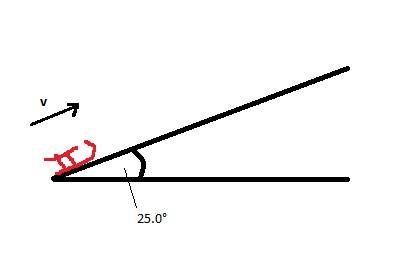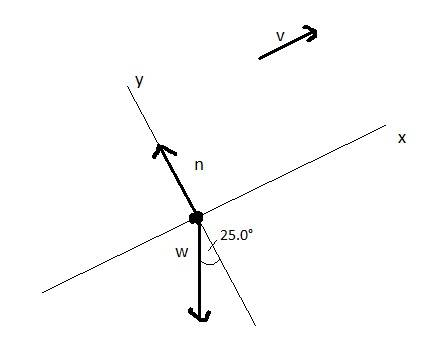# Work-Energy Theorem Exercise

• Argonaut

#### Argonaut

Homework Statement
[Sears and Zemansky's University Physics, 13E] 6.19/(e)
Use the work–energy theorem to solve the problem. Neglect air resistance.
At the base of a frictionless icy hill that rises at 25.0° above the horizontal, a toboggan has a speed of 12.0 m/s toward the hill. How high vertically above the base will it go before stopping?
Relevant Equations
Work-Energy theorem
Kinetic Energy
Trigonometry
My solution is different from the official solution and I don't understand what I did wrong.

Here is my solution:The magnitude of the initial velocity is ##|v_0| = 12.0~\rm{m/s}##, so the vertical component of the initial velocity is ##v_{0-y} = (12.0 \sin{25^{\circ}})~\rm{m/s}##.

Then I use the work-energy theorem and the work equation applicable in case of constant force and straight-line displacement.

##W = \vec{F} \cdot \vec{s}##
and
##W_{tot} = \Delta K = K_1 - K_0 = 0 - K_0 = -\frac{1}{2}v^2m##

Substituting the appropriate vertical variables, I obtain:
$$-mgy=-\frac{1}{2}v_{0-y}^2m \\$$
And then rearranging to make ##y## the subject of the equation I obtain
$$y=\frac{v_{0-y}^2}{2g}=\frac{((12.0 \sin{25^{\circ}})~\rm{m/s})^2}{2(9.80~\rm{m/s^2})} = 1.31~\rm{m}$$

However, the official solution says ##7.35~\rm{m}## which is the value I'd get if I just used the initial velocity magnitude, not its vertical component.

What am I missing?

[Edit: typo in homework statement]

Last edited:

Hi @Argonaut. A couple of points...

1) You have used initial speed = 12.0m/s. But the question (as stated) says it is 12.5m/s.

2) Is the toboggan's weight the only force acting on it?

•MatinSAR and Argonaut
Hi @Argonaut. A couple of points...

1) You have used initial speed = 12.0m/s. But the question (as stated) says it is 12.5m/s.

2) Is the toboggan's weight the only force acting on it?
1) I've made a typo in the statement, so I've edited it for clarity.

2) Right, so I've missed the normal force, haven't I? Let's see how that changes things.

•Steve4Physics
For direct applications of the W-E theorem such as here, it is best to draw a free body diagram with all the forces in place, calculate the work done by each separately, then add them all up to get ##W_{tot}.##

•MatinSAR and Argonaut
What is the initial kinetic energy? What is the final potential energy?

•MatinSAR, Merlin3189 and Argonaut
Thanks, @Chestermiller. I don't yet know what 'potential energy means', but the ##K_i = \frac{1}{2}v^2m## and ##K_f = 0##. So

$$W_{tot} = \Delta K = K_f - K_i = 0 - \frac{1}{2}v_0^2m$$

And then following @kuruman 's advice,So the normal force is perpendicular to the displacement, so it doesn't do any work. The work done by gravity is ##W_{grav} = -w\sin{25^\circ}d = -mg\sin{25^\circ}d##.

Is that correct?

So then I have

$$W_{tot} = W_{grav}$$
$$- \frac{1}{2}v_0^2m = -mg\sin{(25^\circ)}d$$
$$d = \frac{v_0^2}{2g\sin{(25^\circ)}} = \frac{(12.0~\rm{m/s})^2}{2(9.80~\rm{m/s^2})\sin{(25^\circ)}}=17.4~\rm{m}$$

Does this look okay?

And then getting the vertical component of the displacement ##d\sin{(25°)}=7.35~\rm{m}##.

So the ##\sin{(25°)}## term just cancels out. Could I have derived ##y=\frac{(12.0~\rm{m/s})^2}{2(9.80~\rm{m/s^2})}## more easily somehow? Was trigonometry unnecessary to begin with?

And then getting the vertical component of the displacement ##d\sin{(25°)}=7.35~\rm{m}##.

So the ##\sin{(25°)}## term just cancels out. Could I have derived ##y=\frac{(12.0~\rm{m/s})^2}{2(9.80~\rm{m/s^2})}## more easily somehow? Was trigonometry unnecessary to begin with?
Well spotted. There is an alternative trig'-free approach - it requires the use gravitational potential energy as indicated by @Chestermiller in Post #5.

However, the question as stated requires you to use the work-energy theorem so your method is correct.

A couple of points:

1) When writing the kinetic energy expression ##\frac 12 mv^2## it is customary to write the mass first - so it is unconventional to write ##\frac 12 v^2 m##.

2) One form of the work-energy theorem is:

EDIT
work done by net force = change in potential energy.​
Aargh. That should of course be:​
work done by net force = change in kinetic energy.​

Thank you @kuruman.

END EDIT

In this case it is not difficult to realise that the net force is the downhill component of weight, ##mg \sin(25^o)##.

Last edited:
I see, thanks @Steve4Physics . Potential energy is the next chapter so I'll find out soon enough.

And thanks everyone for gently guiding me to the solution. What a great learning experience!

•Steve4Physics
2) One form of the work-energy theorem is:

work done by net force = change in potential energy.
Misleading because if there are non-conservative forces, such as friction, which cannot be derived from a potential, the expression fails. Besides, potential energy does not figure in the W-E theorem formulation.
Incorrect because in the case of conservative forces that can be derived from a potential, by definition the change in potential energy is equal to the negative of the work done by the force. Here, for example the work done by gravity is negative because the force and the vertical displacement are in opposite directions, and the potential energy increases (positive change) as the mass rises farther away from the center of the Earth.
And then getting the vertical component of the displacement ##d\sin{(25°)}=7.35~\rm{m}##.

So the ##\sin{(25°)}## term just cancels out. Could I have derived ##y=\frac{(12.0~\rm{m/s})^2}{2(9.80~\rm{m/s^2})}## more easily somehow? Was trigonometry unnecessary to begin with?
The way to do this by direct application of the W-E theorem, ##\Delta K = W_{tot}##. This strategy is quite simple.
1. Find the total work done by all the forces. That's the right-hand side of the equation.
2. Find the change in kinetic energy. That's the left-hand side of the equation.
3. Set the two equal and find what the question is asking for.

Here is the implementation
1. ##W_{tot}=-mgy##. You found that already. Note that ##y## is the vertical displacement that you are looking for.
2. ##\Delta K=0-\frac{1}{2}mv_0^2##. Here ##v_0=12.5~##m/s
3. ##-mgy=-\frac{1}{2}mv_0^2.##
Note that the incline angle is totally irrelevant here. It would come into play if the problem asked to find how far up the incline the mass will travel before it stops.

Last edited:
••MatinSAR, Argonaut and Steve4Physics
Misleading because if there are non-conservative forces, such as friction, which cannot be derived from a potential, the expression fails.
Yes indeed. Thank you. It was a total 'c*ck-up' (UK terminology). What I meant to say was:

The work-energy theorem can be stated as:

work done by net force = change in kinetic energy​

I had potential energy in the back of my mind and the word 'kinetic' somehow got changed to 'potential'!

•MatinSAR and kuruman
The way to do this by direct application of the W-E theorem, ##\Delta K = W_{tot}##. This strategy is quite simple.
1. Find the total work done by all the forces. That's the right-hand side of the equation.
2. Find the change in kinetic energy. That's the left-hand side of the equation.
3. Set the two equal and find what the question is asking for.

Here is the implementation
1. ##W_{tot}=-mgy##. You found that already. Note that ##y## is the vertical displacement that you are looking for.
2. ##\Delta K=0-\frac{1}{2}mv_0^2##. Here ##v_0=12.5~##m/s
3. ##-mgy=-\frac{1}{2}mv_0^2.##
Note that the incline angle is totally irrelevant here. It would come into play if the problem asked to find how far up the incline the mass will travel before it stops.
Thank you. This is illuminating.